# Addressing modes of 8085 in 8085 Microprocessor

Using mnemonics without any alteration in the content, data can be transferred in three different cases –

• From one register to another register

• From the memory to the register and

• From the register to the memory

These can be guided by addressing modes. Addressing modes in 8085 can be classified into 5 groups −

In this mode, the 8/16-bit data is specified in the instruction itself as one of its operands. For example MVI E, ABH means ABH is copied into register A.

• MVI E ABH

 Before After (A) Any value ABH

As an example, if we consider instruction MVI E, ABH then it means that ABH will be moved or copied to the register E.And, as a result, the previous value of E will get overwritten.

Hex Codes
Mnemonic
Comment
2000
1E
MVI E, ABH
E ← ABH
2001
AB

ABH as operand

This instruction will have seven T-states as shown below.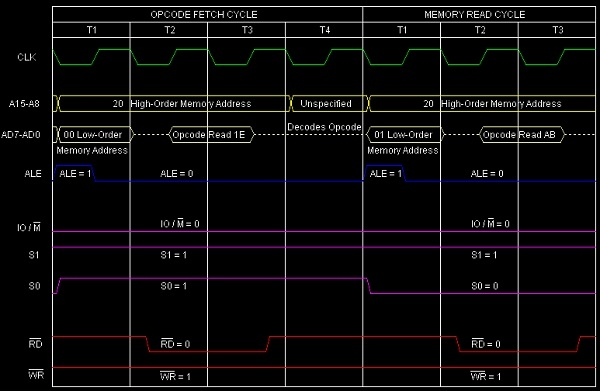Summary - So this instruction MVI E, ABH requires 2-Bytes, 2-Machine Cycles (Opcode Fetch and Memory Read) and 7 T-States for execution as shown in the timing diagram.

In this mode, the data is copied from one register to another. For example, MOV A, B: means data in register B is copied to register A.

• MOV E, H

It occupies only 1-Byte in memory. MOV E, H is an example instruction of this type. It is a 1-Byte instruction. Suppose E register content is AB H, and H register content is 9C H. When the 8085 executes this instruction, the contents of E register will change to 9C H.This is shown as follows.

 Before After (E) ABH 9CH (H) 9CH 9CH

Hex Codes
Mnemonic
Comment
2004
5C
MOV E, H
E ← H

Note that H register’s content has not been changed at all. Although Intel has called it a “move” instruction, but actually, in reality, it seems to be a “copy” instruction.

The timing diagram of MOVE, H instruction is as follows -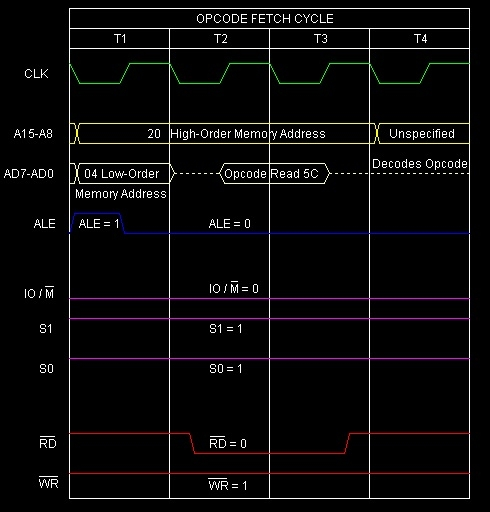Summary - So this instruction MOV E, H requires1-Byte, 1-Machine Cycles (Opcode Fetch) and 4 T-States for execution as shown in the timing diagram.

In this mode, the data is directly copied from the given address to the register. For example LDA 3000H: means the data at address 3000H is copied to register A.

• LDA 4050H

Let us consider LDA 4050 Has an example instruction of this type. It is a 3-Byte instruction. The initial content of memory address 4050H is ABH. initial accumulator content is CDH. As after execution A will be initialized with value ABH. Memory location 4050H will still remain with the content ABH. The results of the execution of this instruction are as below –

Before
After
(4050)
ABH
ABH
A
CDH
ABH

Hex Codes
Mnemonic
Comment
2008
3A
LDA 4050H
A ← Content of the memory location 4050H
2009
50

Low order Byte of the address
200A
40

High order Byte of the address

Here is the timing diagram of the instruction LDA 4050H –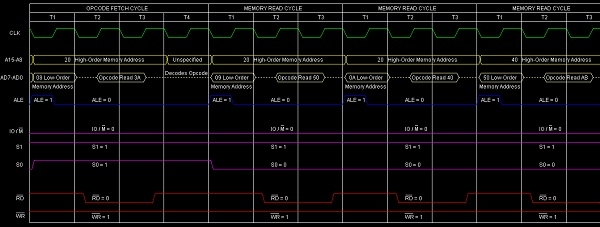Summary - So this instruction LDA 4050H requires 3-Bytes, 4-Machine Cycles (Opcode Fetch, Memory Read, Memory Read, Memory Read) and 13 T-States for execution as shown in the timing diagram.

In this mode, the data is transferred from one register to another by using the address pointed by the register. For example, MOV A, M: means data is transferred from the memory address pointed by the register pair HL to the register A.

• MOV E, M

It occupies only 1-Byte in memory. MOVE, M is an example instruction of this type. It is a 1-Byte instruction. Suppose E register content is DBH, H register content is 40H, and L register content is 50H. Letus say location 4050H has the data value AAH. When the 8085 executes this instruction, the contents of E register will change to AAH, as shown below –

Before
After
(E)
DBH
AAH
(HL)
4050H
4050H
(4050H)
AAH
AAH

Hex Codes
Mnemonic
Comment
2008
2A
MOV E, M
E ← Content of the memory location pointed by HL register pair

The timing diagram for this MOV E, M instruction is as follows –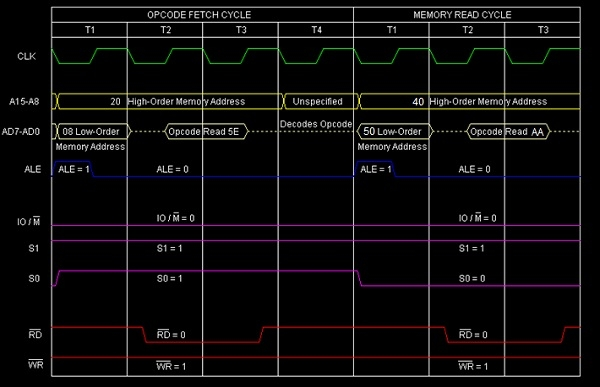Summary - So this instruction MOV E, M requires 1-Byte, 2-Machine Cycles (Opcode Fetch, Memory Read) and 7 T-States for execution as shown in the timing diagram.

This mode doesn’t require any operand; the data is specified by the opcode itself. For example: CMA, CMP.

• CMP E

Let us consider one sample instruction CMPE falling in this category. It is a 1-Byte instruction so during execution of this instruction it will occupy only a single Byte in memory. The result of the execution of this instruction has been depicted with the following set of examples –

## Example 1

Before
After
(A)
50H
50H
(E)
70H
70H
(Temp)
Any value
E0H
(F)
Any values
Cy=1,AC=0,S=1,P=0,Z=0

## Example 2

Before
After
(A)
70H
70H
(E)
50H
50H
(Temp)
Any value
20H
(F)
Any values
Cy=0,AC=0,S=0,P=0,Z=0

## Example 3

Before
After
(A)
50H
50H
(E)
50H
50H
(Temp)
Any value
00H
(F)
Any values
Cy=0,AC=0,S=0,P=1,Z=1

Hex Codes
Mnemonic
Comment
2004
BB
CMP E
Temp = Register A - Register E

The timing diagram against this instruction CMP Execution is as follows –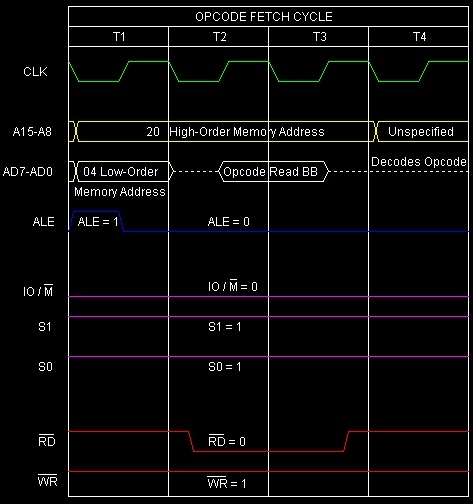Summary - So this instruction CMP E requires 1-Byte, 1-Machine Cycle (Opcode Fetch) and 4 T-States for execution as shown in the timing diagram.